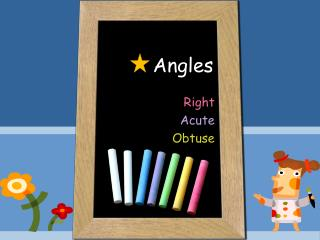# Angles - PowerPoint PPT PresentationDownload PresentationAngles

AnglesDownload Presentation## Angles

- - - - - - - - - - - - - - - - - - - - - - - - - - - E N D - - - - - - - - - - - - - - - - - - - - - - - - - - -
##### Presentation Transcript

1. Angles Right Acute Obtuse

2. TEKS • (6)  Geometry and spatial reasoning. The student uses geometric vocabulary to describe angles, polygons, and circles. The student is expected to: (A)  use angle measurements to classify angles as acute, obtuse, or right

3. Angle • What is a Angle? • - Two rays that share the same endpoint from an angle. The point where the rays intersect is called the vertex of the angle. The two rays are called the sides of the angle.

4. What do we use to help us? A protractor Here is a standard protractor like you use in the classroom.

5. When we use a protractor, we need to line it up correctly. You need to make sure the protractor is lined up correctly. Is this ready to measure the angle?

6. Were you right......................it wasn’t Look for the upside down ‘T’ in the middle of the straight line on your protractor. This needs to be exactly on the vertex of your angle.

7. We need to remember..... It doesn’t matter which way round the angle is, you ALWAYS need to line the upside down ‘T’ to the vertex of the angle.

8. Right Angle • What is a Right Angle? • - an angle whose measure is 90 degrees. This angle is 90 degree. Therefore, it is a Right triangle.

9. Right Angle around Us Can you find right angles?

10. Acute Angle • What is a Acute Angle? • - an angle whose measure is less than 90 degrees. Hmm.. This angle is about 77 degree which is less than 90 degree. Therefore, it is a Acute triangle.

11. Acute Angle around Us Can you find acute angles?

12. Obtuse Angle • What is a Obtuse Angle? • - an angle whose measure is more than 90 degrees. Wow, This angle is 103 degree. Hmm.. This is bigger angle than 90 degree angle. Therefore, it is a Right triangle.

13. Obtuse Angle around Us Can you find obtuse angles?

14. Reference • http://themarketingguy.wordpress.com/2007/06/14/ • http://www.mathsisfun.com/angles.html • www.freephotobank.com • http://www.baskinrobbins.com • http://www.faqs.org/photo-dict/phrase/1274/waffle.html • http://www.babygadget.net/robotA280.jpg • http://www.blueberryforest.com/images/Images_haba/haba-milk-carton-mini-271x392.jpg • http://image.misterart.com/grouppix/528x352/2000/g2025.jpg • http://img.timeinc.net/recipes/i/recipes/sl/03142008/cheesecake-sl-520368-l.jpg • http://www.tenthousandvillages.com/nav/product/zoom_6600360.jpg • http://www.electricpig.co.uk/wp-content/uploads/2008/11/ep_sandwich.jpg • http://www.fotosearch.com/bthumb/SUE/SUE104/BWBW0867.jpg • http://www.catawbaschools.net/schools/BallsCreek/Images1/pizza.jpg • http://img.alibaba.com/photo/101176672/Model_Planes_Rc_Model_Rc_Hobby_Rc_Toy_Rc_Plane_Rc_Airplane.jpg • http://www.brake.org.uk/resources/images/alex%20letter%20A.jpg